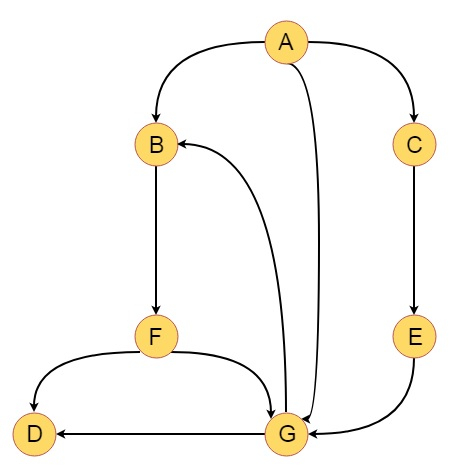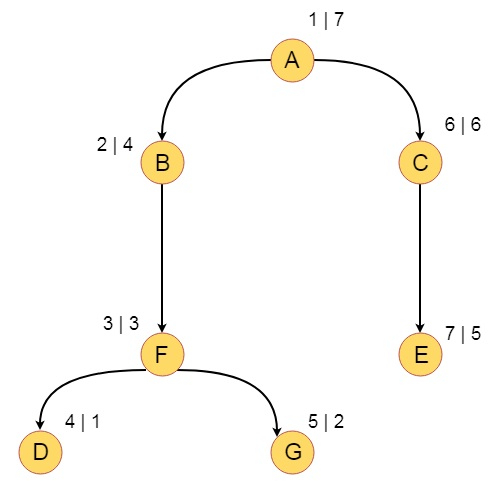# Depth-First Search on a Digraph in Data Structure

The Depth first search for graphs are similar. But for Digraphs or directed graphs, we can find some few types of edges. The DFS algorithm forms a tree called DFS tree. There are four types of edges called −

• Tree Edge (T) − Those edges which are present in the DFS tree

• Forward Edge (F) − Parallel to a set of tree edges. (From smaller DFS number to larger DFS number, and Larger DFS completion number to Smaller DFS completion number)

• Backward Edge (B) − From larger DFS number to Smaller DFS number and Smaller DFS completion number to Larger DFS completion number.

• Cross Edge (C) − Larger DFS number to Smaller DFS number, and Larger DFS completion number to Smaller DFS completion number.

Suppose we have a graph like below −Now we will perform DFS by taking A as initial vertex, and put the DFS number and DFS completion numbers. So the tree will be look like below −So the DFS traversal is A, B, F, D, G, C, E

The Tree edges are − T = {(A, B), (B, F), (F, D), (F, G), (A, C), (C, E)}

The Forward edges are − F = {(A, G)}

The Backward edges are − B = {(G, B)}

The Cross edges are −C = {(G, D)}